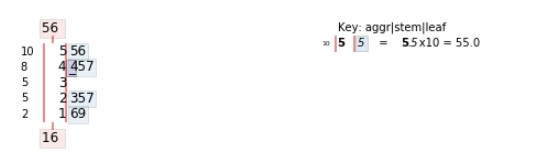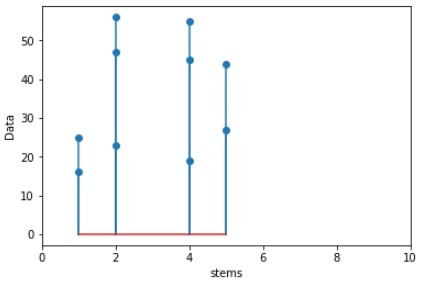# Stem and Leaf Plots in Python

Stem and Leaf Plot is a way of representing the data. This plot is used to show the absolute frequency in different classes similar to the frequency distribution table or a histogram. It presents the quantitative data in the graphical format, and the stem-and-leaf plot of quantitative data is said as textual graph as that presents the data according to their most significant numeric digit. Stem and Leaf Plot graph is mainly suitable for smaller data sets.

Stem-and-leaf plot is a tabular presentation where each data value is split into a “stem” (the first digit or digits) and a “leaf” (usually the last digit).

Interpretations:

```"17" is split into "1" (stem) and "7" (leaf)
"69" is split into "6" (stem) and "9" (leaf)```

Procedure to make stem-and-leaf plot:

1. Separate each observation/data into a stem which will consist of all except rightmost digit and leaf, the rightmost digit.
2. Leaf must have only one digit while stem can have as many digits as possible.
3. Write the stem in a vertical column with smallest at the top(but in Python, you will get largest at the top) then draw a vertical line by the right of this column.
4. Write each corresponding leaf in the row to the right of its stem just after the vertical line, in ascending order out from the stem.

Example:

Let’s say there are 10 Technical Content Writers at GeeksforGeeks. Each of them submitted 100 articles
to publish at the site. Out of 100 articles, the number of articles which had some errors are given below for each 10 content writers –

`16, 25, 47, 56, 23, 45, 19, 55, 44, 27`

Stem-and-leaf plot will be –

```1 | 69
2 | 357
4 | 457
5 | 56```

Plot in Python using stemgraphic module –
To plot stem-and-leaf plot in Python, we need to install the <`strong>stemgraphic module`. You can install stemgraphic module from Jupyter Notebook as –

```import sys
!{sys.executable} -m pip install stemgraphic
```

Below is the code –

 `# importing the module ` `import` `stemgraphic ` ` `  `data ``=` `[``16``, ``25``, ``47``, ``56``, ``23``, ``45``, ``19``, ``55``, ``44``, ``27``] ` ` `  `# calling stem_graphic with required parameters, ` `# data and scale ` `stemgraphic.stem_graphic(data, scale ``=` `10``) `

Output:Explanation –
The leftmost column in the above plot is the frequency count. There are two observations in the range 10-20 and 3 observations in the range 20-30, which gives total of 5 observations in the range 0-30. Continuing in the same way, there is total of 10 observations which is at the top in the same column. Then after a vertical line, there are two values, one at bottom most we have 16. While at the topmost we have 56, these values are nothing but the minimum and maximum values respectively in the given data set. After that, we have the stem values and following that we have leaves values separating stem by the vertical line.

Note: You can install this matplotlib library from Jupyter Notebook as –

```import sys
!{sys.executable} -m pip install matplotlib```

Using `matplotlib.pyplot.stem`

 `# import matplotlib.pyplot library ` `import` `matplotlib.pyplot as plt ` ` `  `data ``=` `[``16``, ``25``, ``47``, ``56``, ``23``, ``45``, ``19``, ``55``, ``44``, ``27``] ` ` `  `# separating the stem parts ` `stems ``=` `[``1``, ``1``, ``2``, ``2``, ``2``, ``4``, ``4``, ``4``, ``5``, ``5``] ` ` `  `plt.ylabel(``'Data'``)   ``# for label at y-axis ` ` `  `plt.xlabel(``'stems'``)   ``# for label at x-axis ` ` `  `plt.xlim(``0``, ``10``)   ``# limit of the values at x axis ` ` `  `plt.stem(stems, data)   ``# required plot `

Output:Reference: https://pypi.org/project/stemgraphic/

My Personal Notes arrow_drop_upCheck out this Author's contributed articles.

If you like GeeksforGeeks and would like to contribute, you can also write an article using contribute.geeksforgeeks.org or mail your article to contribute@geeksforgeeks.org. See your article appearing on the GeeksforGeeks main page and help other Geeks.

Please Improve this article if you find anything incorrect by clicking on the "Improve Article" button below.

Article Tags :

1

Please write to us at contribute@geeksforgeeks.org to report any issue with the above content.